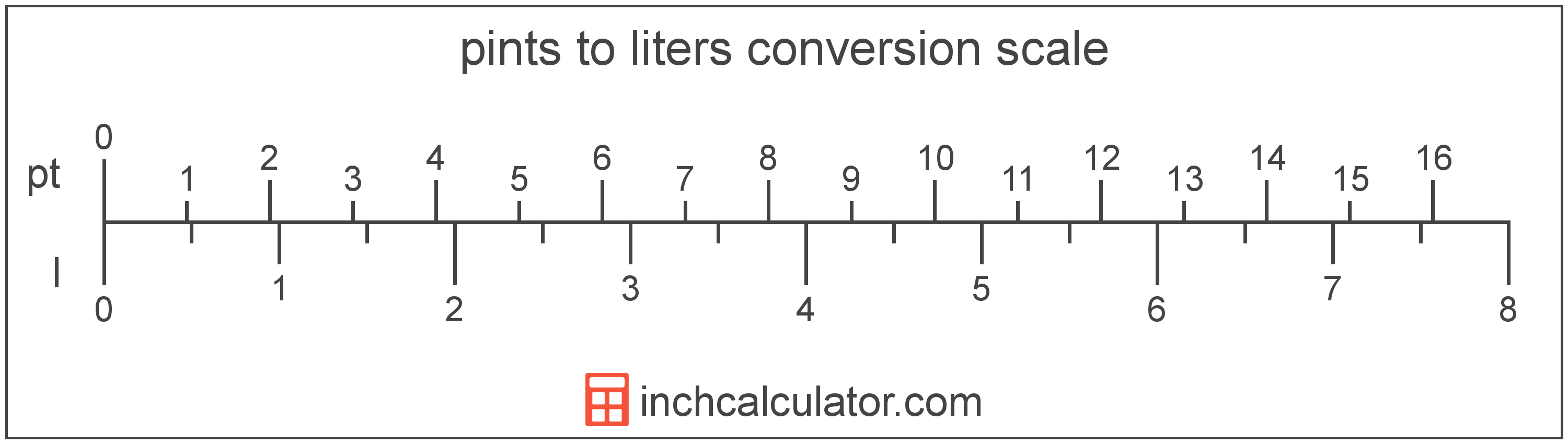# Pints to Liters Conversion

Enter the volume in pints below to get the value converted to liters.

Results in Liters:1 pt = 0.473176 l
Do you want to convert liters to pints?

## How to Convert Pints to LitersTo convert a pint measurement to a liter measurement, multiply the volume by the conversion ratio.

Since one pint is equal to 0.473176 liters, you can use this simple formula to convert:

liters = pints × 0.473176

The volume in liters is equal to the pints multiplied by 0.473176.

For example, here's how to convert 5 pints to liters using the formula above.
5 pt = (5 × 0.473176) = 2.365882 l

## Pints

The US liquid pint is a unit of fluid volume equal to one-eighth of a gallon, one-half of a quart, or two cups. The liquid pint should not be confused with the dry pint (US) or the imperial pint, which are different units.

The pint is a US customary unit of volume. Pints can be abbreviated as pt; for example, 1 pint can be written as 1 pt.

## Liters

A liter is a unit of volume equal to 1,000 cubic centimeters. The liter is a special name defined for the cubic decimeter and is exactly equal to the volume of one cubic decimeter.

The liter is an SI accepted unit for volume for use with the metric system. A liter is sometimes also referred to as a litre. Liters can be abbreviated as l, and are also sometimes abbreviated as L or . For example, 1 liter can be written as 1 l, 1 L, or 1 ℓ.

## Pint to Liter Conversion Table

Pint measurements converted to liters
Pints Liters
1 pt 0.473176 l
2 pt 0.946353 l
3 pt 1.4195 l
4 pt 1.8927 l
5 pt 2.3659 l
6 pt 2.8391 l
7 pt 3.3122 l
8 pt 3.7854 l
9 pt 4.2586 l
10 pt 4.7318 l
11 pt 5.2049 l
12 pt 5.6781 l
13 pt 6.1513 l
14 pt 6.6245 l
15 pt 7.0976 l
16 pt 7.5708 l
17 pt 8.044 l
18 pt 8.5172 l
19 pt 8.9904 l
20 pt 9.4635 l
21 pt 9.9367 l
22 pt 10.41 l
23 pt 10.88 l
24 pt 11.36 l
25 pt 11.83 l
26 pt 12.3 l
27 pt 12.78 l
28 pt 13.25 l
29 pt 13.72 l
30 pt 14.2 l
31 pt 14.67 l
32 pt 15.14 l
33 pt 15.61 l
34 pt 16.09 l
35 pt 16.56 l
36 pt 17.03 l
37 pt 17.51 l
38 pt 17.98 l
39 pt 18.45 l
40 pt 18.93 l

## References

1. National Institute of Standards and Technology, Units outside the SI, https://physics.nist.gov/cuu/Units/outside.html